# Is NH4+ an acid or base? Weak or Strong - Conjugate base

Home  > Chemistry Article > Is NH4 an acid or base?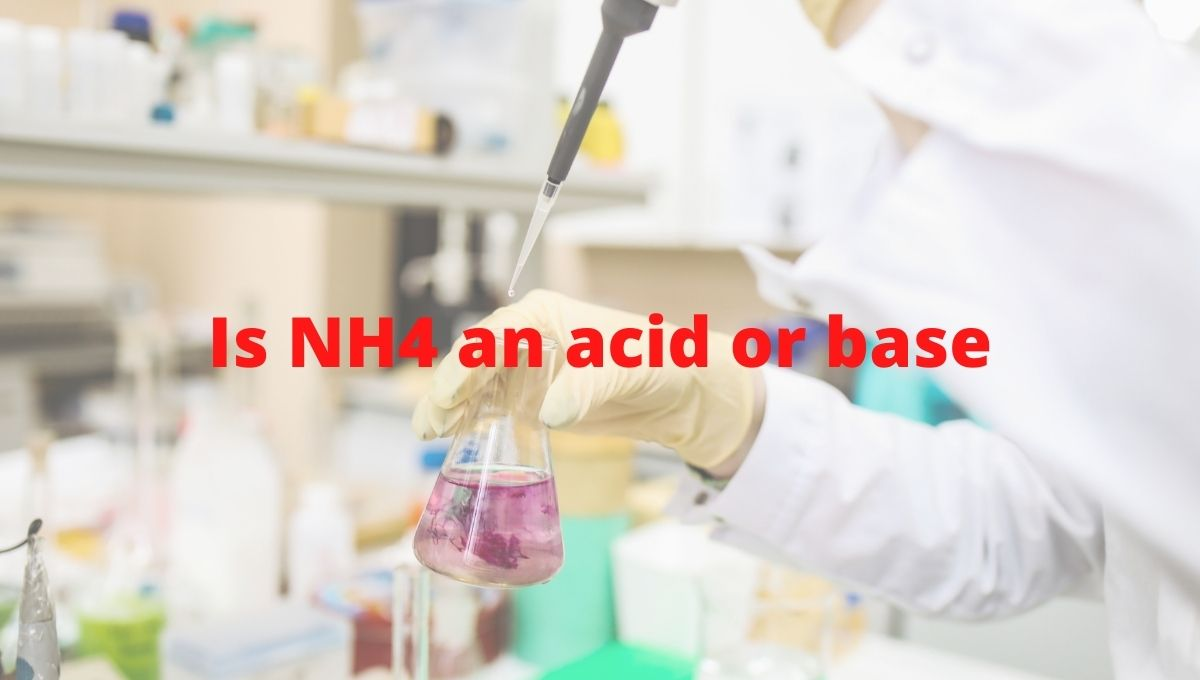Ammonium ion having chemical formula NH4+ is an inorganic compound formed from the protonation of ammonia. It is also called ammonium cation as its structure includes positively charged tetrahedral nitrogen.

Ammonium ions are a waste product of the metabolism of animals. It is toxic to most of the crop species but it is an important source of nitrogen for those species which growing on hypoxic soils.

Simple salts of ammonium ions are soluble in water. In this article, we will discuss Is ammonium ion (NH4+) an acid or base? its conjugate pairs, nature of its strength, etc.

So, Is NH4+ an acid or base? NH4+ is an acidic in nature as it releases the proton when dissolved in an aqueous solution. However, it is a very weak acid having a pH value of 5.5 i.e. near to neutral in the acid-base scale. The proton donating ability of NH4+ makes it mildly acidic.

 Name of Molecule Ammonium ion Chemical formula NH4+ Molar mass 18.039 g/mol Conjugate base Ammonia(NH3) Nature Weak acid Acidity (pKa) 9.25
Page Contents

## Why NH4+ is an acid in nature?

When ammonia reacts with proton donors like H+ or H3O+, it formed an ammonium ion.

⇒ H+ + NH3 → NH4+

And when ammonium ion reacts with proton acceptor like OH, it returns to the neutrally charged ammonia molecule.

⇒ NH4+ + OH → H2O + NH3

The ammonium ion has the ability to donate the proton because there is no lone pair present on the central atom in the NH4+ molecule which can be used to accept the proton from other compounds. Thus, the only ability left for ammonium ion is “donating the proton”.

Whereas NH3 molecule has lone pair on the central atom that can be used to accept the proton and as per Bronsted-Lowry base “a base is the substance that accepts the protons from other species”.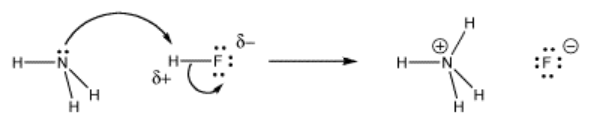Image source:

An acid is something that produces protons when dissolved in an aqueous solution. So, when ammonium ion dissolved in an aqueous solution, it split into two ions (NH3 and H+).

⇒ NH4+ → NH3 + H+

We have two most important acid-base theories to check whether NH4+ is an acid or base in nature.

(a). Arrhenius theory

(b). Bronsted-Lowry theory

Arrhenius theory:-

This theory tells if any compound can release the proton when dissolved in an aqueous solution, qualify as acid and if any compound can release the hydroxide ions when dissolved in an aqueous solution, qualify as the base.

So, in the case of ammonium ion, when dissolved in water, it is ready to “give off” the proton. Hence, we can say, NH4+ is an Arrhenius acid.

Bronsted-Lowry theory:-

This theory tells if any compound can donate the proton to other species and forms a conjugate base, qualify as acid. And for base nature, it’s vice-versa.

So, when NH4+ reacts with hydroxide ion, it donates the one proton to OH and forms a conjugate base(NH3).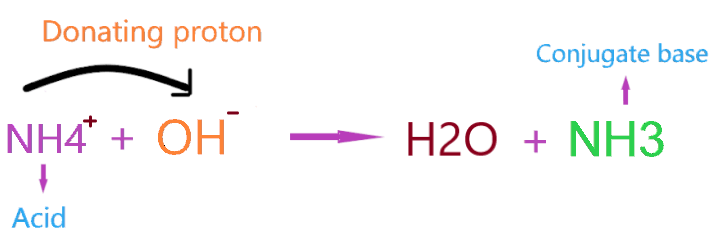As per the above reaction, NH4+ act as Bronsted-Lowry acid and release a proton to become NH3, and OH acts as Bronsted-Lowry base as it accepts the proton and forms H2O.

## Is NH4+ (Ammonium ion) is strong or weak acid?

This part is really confusing to figure out what type of nature an ammonium ion can adopt “strong or weak”. To understand this we should dive into the concept of strong or weak acids.

A strong acid is an acid that tends to dissociate fully or 100% ionized in a solution leaving no parts of it undissociated in solution. Example:- HNO3, HCl, H2SO4, HBr, etc.

A weak acid is an acid that is only partially dissociated in a solution means some of the acids remain undissociated in the solution. Example:- CH3COOH, H3PO4, HCN, HCOOH, H2CO3, HF, etc.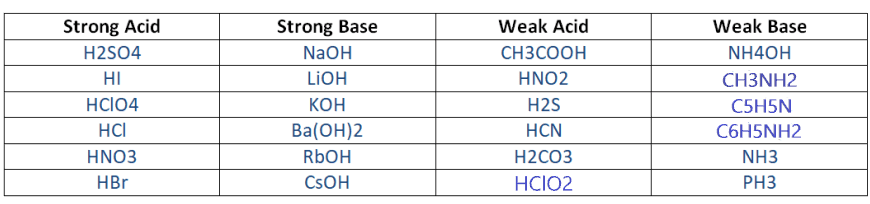Now come to the part Is NH4+ a strong or weak acid? NH4+ is a weak acid as it doesn’t tend to dissociate 100% in an aqueous solution. It has a very small value of acid dissociation constant(Ka).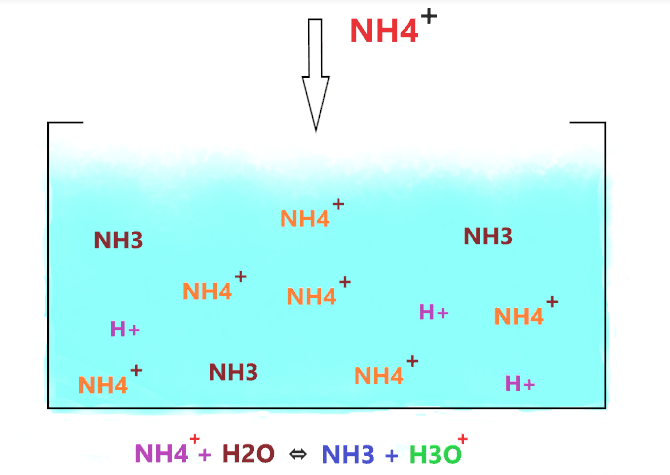As shown in the figure, when NH4+ is dissolved in water, then it produces two products(NH3 and H+), but the thing is, NH3 is a weak base that accepts the hydrogen ions in water and again forms the NH4+, therefore, the favor of reaction lies to the left side.

So, not all the NH4+ molecules dissociate, most of them stay together and only a few of them dissociate and liberate H+ ions. And those NH4+ dissociate into ions, their most of the split ions(NH3 and H+) reform with each other and again form the NH4+ molecule.

“The acid dissociation constant value is used to distinguish the strong acid from weak acid”. The higher the value of Ka, the more the acid dissociates.

Let’s understand why NH4+ acts as the weak acid with the help of the dissociation constant value concept.

⇒ If the value of the dissociation constant of acid is greater than 1 (Ka > 1), then the nature of the compound is a strong acid.

⇒ If Ka < 1, then the nature of the compound is a weak acid.

⇒ If Kb >1, then the nature of the compound is a strong base.

⇒ If Kb <1, then the nature of the compound is a weak base.

To figure out the dissociation constant value of NH4+, first, we should know the Kb value for NH3.

∴ The dissociation constant value(Kb) for ammonia is 1.8 × 10-5.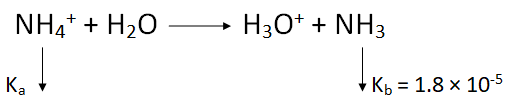∴ Ka = Kw/Kb

⇒ Ka = 10-14/ 1.8 × 10-5             [∴ Kw = 10-14]

∴ Ka for ammonium ion = 5.6 × 10-10

The value of Ka for NH4+ is way lower than 1, therefore, NH4+ is a weak acid in nature.

## Is NH4+ a Lewis acid or base?

Lewis acid is simply acids that have the tendency of “accepting the pair of electrons” whereas Lewis base has a tendency of “donating the pair of electrons”.

⇒ Lewis acid → electron pair acceptor

⇒ Lewis base → electron pair donator

So, Is NH4+ a lewis acid or base? NH4+ is neither lewis acid nor lewis base because no lone pair of electrons is present on the central atom of NH4+, so it doesn’t have the ability to donate the lone pair to qualify as Lewis base.

Also, NH4+ has a full octet means it doesn’t have enough space to accept the lone pair of the electron from other compounds to qualify as Lewis acid.

A full octet means having a 8 electrons in the valence shell of an atom. In NH4+ molecule, “nitrogen has 5 valence electron and sharing four more electrons with hydrogen atoms, also it loses one electron from a positive charge present on it. (5 + 4 – 1 = 8 electrons)

## What is the Conjugate base of NH4+

As we know, an ammonium ion is a weak acid that loses the proton when reacts with water and forms a conjugate base as per the theory of Bronsted-Lowry.

From this theory, we got to know when an acid loses one proton from itself, it makes a conjugate base. So, we can say, “A conjugate base is a base which formed when an acid loses a hydrogen ion or proton.

The conjugate base of NH4+ is ammonia (NH3) because the ammonium ion loses one proton when reacted with the water molecule(H2O).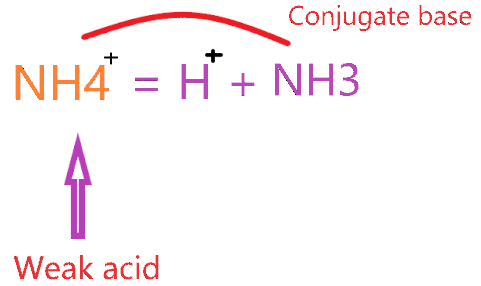## Which is more stable NH4+ or NH3?

Always remember (A neutral molecule is more stable than any charged molecule). So, we can say from NH4+ and NH3, NH3 is more stable as it doesn’t have any charge on it.

But the stability of a molecule depends on the medium i.e. Alkaline or Acidic.

In an acidic environment, NH4+ is more stable than NH3 and in an alkaline environment, NH3 is more stable than NH4+ because NH3 is a weak base in nature and NH4+ is a weak acid.

If there is no medium mention then an uncharged molecule is always more stable than a charged molecule.

## Properties of Ammonium

• It has a molar mass of 18.039 g·mol−1.
• The molecular shape of NH4+ is tetrahedral.
• Simple salts of NH4+ are soluble in water.
• At high pressure, it behaves as metal.
• Under normal conditions, ammonium exists as amalgam.

## Conclusion

Ammonium is formed when NH3 accepts the proton and forms a dative covalent bond with hydrogen. It is a polyatomic ion with a positive charge and contains no lone pair of electrons. In this article, we discussed the acid-base nature of NH4+

• The pKa value of ammonium is 9.25.
• The conjugate base of NH4+ is ammonia(NH3).
• Is ammonium (NH4+) an acid or base? NH4+ is acidic in nature having a pH value close to 5.5. When dissolved in an aqueous solution it releases the one proton and comes to in stable form(NH3).
• NH4+ is a weak acid because it partially dissociates in an aqueous solution and the acid dissociation value of it (Ka) is very less than 1 which considered a weak acidic value.
• NH4+ is neither lewis acid nor lewis base as it doesn’t have the ability to “accepting the lone pair of electrons” and “donating the lone pair of electrons”.
##### Subscribe to Blog via Email

Join 2 other subscribers

Share it...